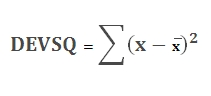# SQL Server deviation squared function

Updated: 6 August 2010

Use DEVSQ _q to calculate the sum of the squares of deviations of data points from their sample mean. The equation for deviation squared isSyntax
SELECT [wctStatistics].[wct].[DEVSQ_q] (
<@Known_x_RangeQuery, nvarchar(4000),>)
Arguments
@Known_ x_RangeQuery
the select statement, as text, used to determine the known x-values to be used in the DEVSQ_q calculation.
Return Types
float
Remarks
·         No GROUP BY is required for this function even though it produces aggregate results.
·         For simpler queries, consider using the DEVSQ function.
Examples
To determine the deviation squared for all students in all subjects:

This produces the following result

----------------------
2236.75490545455

(1 row(s) affected)

To calculate the deviation squared for each subject

SELECT Distinct s.subject
,wct.DEVSQ_q('Select grade from #s1 where #s1.subject = ' + char(39) + s.subject + char(39))
from #s1

This produces the following result

subject
-------------------------------------------------- ----------------------
Foreign Language                                   553.967036363636
History                                            166.494695454545
Literature                                         166.494695454545
Math                                               360.071127272727
Science                                            254.2028

(5 row(s) affected)

To calculate the deviation squared for each student

SELECT distinct s.student
,wct.DEVSQ_q('Select grade from #s1 where student = ' + char(39) + s.student + char(39))
from #s1 s

This produces the following result

student
-------------------------------------------------- ----------------------
Student 01                                         1.77212000000002
Student 02                                         7.96292
Student 03                                         11.7302
Student 04                                         14.94752
Student 05                                         17.74432
Student 06                                         20.39848
Student 07                                         23.00088
Student 08                                         25.52052
Student 09                                         27.96032
Student 10                                         30.44448
Student 11                                         33.0344
Student 12                                         35.7018
Student 13                                         38.39332
Student 14                                         41.27792
Student 15                                         44.3702
Student 16                                         47.7230800000001
Student 17                                         51.2705199999999
Student 18                                         55.4961200000001
Student 19                                         60.2548799999999
Student 20                                         66.1875200000001
Student 21                                         74.28668
Student 22                                         87.2487200000002

(22 row(s) affected)

### SupportCopyright 2008-2023 Westclintech LLC         Privacy Policy        Terms of Service